# EEL 5525 Practice Exam #1

EEL 5525 Practice Exam #1

Fall 2011

Lectures 1-7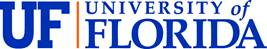# Instructions:

Complete the exam on the space provided. When time is called, stop all work and follow the instructions provided. Any work that is not collected when called for will not be graded. The honor system will be strictly enforced.

Allowed

Open book

Open notes

Pocket calculators and laptops

Figures and tables

Disallowed

Unauthorized Internet access

Cell phones

Q1: Sampling Theorem and Quantization

You are to analyze the audio recording and playback system shown below. The input audio frequency range is fÎ[0, 3.5] kHz. The listener’s hearing range is fÎ[0, 8] kHz. The ADC operates at the programmable sample rate of fs = n8kHz, n an integer.

 ²a)    What is the lowest sampling frequency fs that will insure that the original audio signal x(t) can be (theoretically) reconstructed from its time-series samples x[k], without aliasing?

b)    The room is presumed quiet and you begin recording at a sample rate of fs=8k Sa/s. When played back you hear a 2k Hz “buzzing sound” in the captured signal.  What is the expected minimum frequency of the extraneous tone that could have created this effect?

c)    You decide to place an ideal analog lowpass anti-aliasing filter in front of the ADC. What should be the filter’s passband cut-off frequency?

d)    The signed ±10V ADC provides an 8-bit output with an input |x(t)|<10V.  What is the ADC’s quantization step size?

e)    The signed 8-bit ADC’s output is sent to an accumulator that produces an output given by: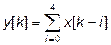(not a MAC)

What is the minimum number of integer bits that must be assigned to the accumulator to insure overflow-free run-time performance?

Q.2:z-Transforms

 Table 1: Primitive Signals and their z-Transform Time-domain z-transform d[k] 1 u[k] z/(z–1) aku[k] z/(z–a) kaku[k] az/(z–a)2

You are studying a causal signal x[k] having a z-transform X(z)= (z+1)2/(z-1)(z-0.5)2. The signal has a Heaviside expansion given by:

X(z)= (z+1)2/((z-1)(z-0.5)2 ) = A + Bz/(z-1) + Cz/(z-0.5) + Dz/(z-0.5)2.

Invert X(z) (Hint:  Think X(z) = X(z)/z).

a)    What is A?

b)    What is B?

c)  What is C?

d)  What is D?

e)  What is x[k]?

f)  What is x[¥]?

g)  What is x?

# 3.  Sampling and Data Conversion:

A real signal x(t) = sin(2p(103)t) + sin(2p(6*103)t) (f1=1kHz, f2=6kHz) is presented to the system shown below.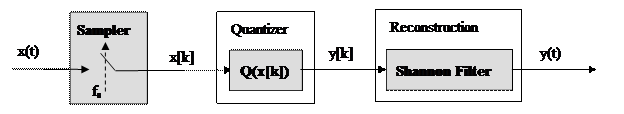a- What is the Nyquist sampling rate (Sa/s)?

b- If x(t) is sampled at a rate fs=8kHz, what is the reconstructed signal in the form y(t) = A sin(2pf1t) + B sin(2pf2t)? (Assume the quantizer is bypassed, that is let x[k]=y[k])

c- The qunatizer is in-place. The resulting signed 8-bit ADC having a ±8 volt dynamic range quantizes the input an analog signal bounded by |x(t)|£ 5 volts. What is the ADC’s quantization step size in volts/bit?

d- What is the statistical quantization error in bits (i.e., how many fractional bits are statistically preserved)?

4: Sampling Theorem and Quantization]

The home edition of American Idol uses the recording system shown below.  The ADC is sampled at a 12000 Sa/s rate.  The human vocal input is assumed limited to 4 kHz.a. The sample rate is chosen to be 12k Sa/s. To test the system, a hand-held audio signal generator is placed near the microphone.  The signal generator produces a sinusoid tone x(t)=sin(2pf0t) where f0 = 8kHz.  What is the reconstructed signal y(t)?b.  The signal generator’s frequency is set to f0 = 4 kHz but the gain on the electronic signal generator, used in Part 1.b, is set too high and produces a square wave x(t) = sign(sin(2pf0t)) having a Fourier series representation given by:]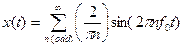Assume that x(t) can be essentially model using only the 1st, 3rd, and 5th harmonics having amplitudes a1= 2/p, a3= 2/3p, and a5= 2/5p respectively, where f0 = 4 kHz and fs = 12 kSa/s. What is the reconstructed output signal y(t)?

5: Discrete-time system

Consider the non-causal discrete-time systems shown below.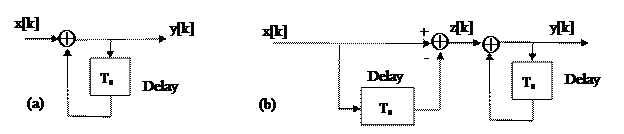The difference equation that applies to the system shown on the left of the Figure shown above is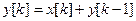.

a. – Is the system BIBO stable?

b.- What is the difference equation the applies to the system shown on the right of the Figure shown above?

c.-  What are the system’s first 4 outputs if y[-1]=0 (system at-rest) and x[k]=u[k] (unit step)?

Attachments:EEL 5525 Practice Exam #1

Fall 2011

Lectures 1-7

# Instructions:

Complete the exam on the space provided. When time is called, stop all work and follow the instructions provided. Any work that is not collected when called for will not be graded. The honor system will be strictly enforced.

Allowed

Open book

Open notes

Pocket calculators and laptops

Figures and tables

Disallowed

Unauthorized Internet access

Cell phones

Q1: Sampling Theorem and Quantization

You are to analyze the audio recording and playback system shown below. The input audio frequency range is fÎ[0, 3.5] kHz. The listener’s hearing range is fÎ[0, 8] kHz. The ADC operates at the programmable sample rate of fs = n8kHz, n an integer.

 ²
1. What is the lowest sampling frequency fs that will insure that the original audio signal x(t) can be (theoretically) reconstructed from its time-series samples x[k], without aliasing?

1. The room is presumed quiet and you begin recording at a sample rate of fs=8k Sa/s. When played back you hear a 2k Hz “buzzing sound” in the captured signal.  What is the expected minimum frequency of the extraneous tone that could have created this effect?

1. You decide to place an ideal analog lowpass anti-aliasing filter in front of the ADC. What should be the filter’s passband cut-off frequency?

1. The signed ±10V ADC provides an 8-bit output with an input |x(t)|<10V. What is the ADC’s quantization step size?

1. The signed 8-bit ADC’s output is sent to an accumulator that produces an output given by:

(not a MAC)

What is the minimum number of integer bits that must be assigned to the accumulator to insure overflow-free run-time performance?

Q.2: z-Transforms

 Table 1: Primitive Signals and their z-Transform Time-domain z-transform d[k] 1 u[k] z/(z–1) aku[k] z/(z–a) kaku[k] az/(z–a)2

You are studying a causal signal x[k] having a z-transform X(z)= (z+1)2/(z-1)(z-0.5)2.  The signal has a Heaviside expansion given by:

X(z)= (z+1)2/((z-1)(z-0.5)2 ) = A + Bz/(z-1) + Cz/(z-0.5) + Dz/(z-0.5)2.

Invert X(z) (Hint:  Think X(z) = X(z)/z).

1. What is A?

1. What is B?

1. c) What is C?

1. d) What is D?

1. e) What is x[k]?

1. f) What is x[¥]?

1. g) What is x?

# 3.  Sampling and Data Conversion:

A real signal x(t) = sin(2p(103)t) + sin(2p(6*103)t) (f1=1kHz, f2=6kHz) is presented to the system shown below.

a- What is the Nyquist sampling rate (Sa/s)?

b- If x(t) is sampled at a rate fs=8kHz, what is the reconstructed signal in the form y(t) = A sin(2pf1t) + B sin(2pf2t)? (Assume the quantizer is bypassed, that is let x[k]=y[k])

c- The qunatizer is in-place. The resulting signed 8-bit ADC having a ±8 volt dynamic range quantizes the input an analog signal bounded by |x(t)|£ 5 volts. What is the ADC’s quantization step size in volts/bit?

d- What is the statistical quantization error in bits (i.e., how many fractional bits are statistically preserved)?

4: Sampling Theorem and Quantization]

The home edition of American Idol uses the recording system shown below.  The ADC is sampled at a 12000 Sa/s rate.  The human vocal input is assumed limited to 4 kHz.

1. The sample rate is chosen to be 12k Sa/s. To test the system, a hand-held audio signal generator is placed near the microphone. The signal generator produces a sinusoid tone x(t)=sin(2pf0t) where f0 = 8kHz. What is the reconstructed signal y(t)?

1. The signal generator’s frequency is set to f0 = 4 kHz but the gain on the electronic signal generator, used in Part 1.b, is set too high and produces a square wave x(t) = sign(sin(2pf0t)) having a Fourier series representation given by:]

Assume that x(t) can be essentially model using only the 1st, 3rd, and 5th harmonics having amplitudes a1= 2/p, a3= 2/3p, and a5= 2/5p respectively, where f0 = 4 kHz and fs = 12 kSa/s. What is the reconstructed output signal y(t)?

5: Discrete-time system

Consider the non-causal discrete-time systems shown below.

The difference equation that applies to the system shown on the left of the Figure shown above is .

1. – Is the system BIBO stable?

b.- What is the difference equation the applies to the system shown on the right of the Figure shown above?

c.-  What are the system’s first 4 outputs if y[-1]=0 (system at-rest) and x[k]=u[k] (unit step)?